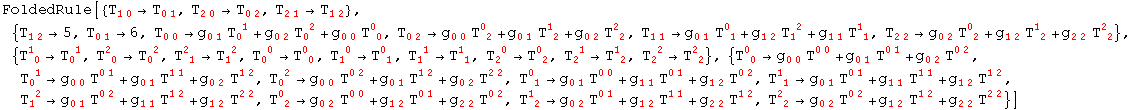7.8. Replacing TensorValues in expressions

ToValues        Replace tensor values in a given expression

Replacing TensorValues in expressions

As we have seen, xCoba` does not provide an automatic tool for setting values (use Set or SetDelayed instead of rules), because this is potentially dangerous and removing the definitions would not be trivial. However, it is very simple to apply all the rules in TensorValues to a given expression

In:=In:=Out=In:=In:=Out=In:=Out=In:=Out=That expression has only the tensor T and so we get

In:=Out=Now the metric tensors are explicit and hence:

In:=Out=Created by Mathematica  (May 16, 2008)